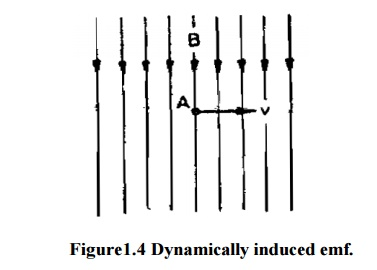Home | | Electrical Machines I | Statically and Dynamically Induced Emf

# Statically and Dynamically Induced Emf

Induced electro motive forces are of two types. They are, i) Dynamically induced emf. ii) Statically induced emf .

Statically And Dynamically Induced Emf.

Induced electro motive forces are of two types. They are,

i)                Dynamically induced emf.

ii)             Statically induced emf .

## 1. Statically Induced Emf

Statically Induced emf is of two types. They are

1 .Self induced emf

2. Mutually induced emf.

## 1.1 Self Inductuced emf

Self induction is that phenomenon where by a change in the current in a conductor induces an emf in the conductor itself. i.e. when a conductor is given current, flux will be produced, and if the current is changed the flux also changes, as per Faraday's law when there is a change of flux, an emf will be induced. This is called self induction. The induced emf will be always opposite in direction to the applied emf. The opposing emf thus produced is called the counter emf of self induction.

Uses of Self induction

.1.  In the fluorescent tubes for starting purpose and to reduce the voltage.

2.   In regulators, to give reduced voltage to the fans.

3.   In lightning arrester.

4.   In auto- transformers.

5.   In smooth choke which is used in welding plant.

## 1.2 Mutually Induced EMF

It is the electromagnetic induction produced by one circuit in the near by second circuits due to the variable flux of the first circuit cutting the conductor of the second circuit, that means when two coils or circuits are kept near to each other and if current is given to one circuit and it is changed, the flux produced due to that current which is linking both the coils or circuits cuts both the coils, an emf will be produced in both the circuits. The production of emf in second coil is due to the variation of current in first coil known as mutual induction.

### Uses:

1.              It is used in ignition coil which is used in motor car.

2.              It is also used in inductance furnace.

3.              It is used for the principle of transformer

## 2. Dynamically induced EMF

Dynamically induced emf means an emf induced in a conductor when the conductor moves across a magnetic field. The Figure shows when a conductor “A”with the length “L” moves across a “B” wb/m2.Flux density with “V” velocity, then the dynamically induced emf is induced in the conductor. This induced emf is utilized in the generator. The quantity of the emf can be calculated using the equation

emf= Blv volt

Study Material, Lecturing Notes, Assignment, Reference, Wiki description explanation, brief detail
Electrical machines : Magnetic Circuits and Magnetic Materials : Statically and Dynamically Induced Emf |